# Unity Shader 公告板， billborad的实现思路们--------------马上发车，去往东莞的路上，你我不在孤独

transform.LookAt(Camera.main.transform);

transform.rotation = Camera.main.transform.rotation;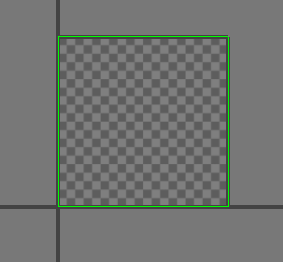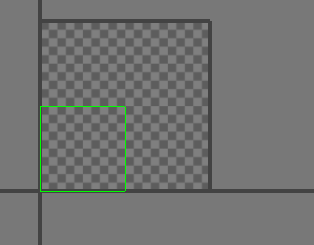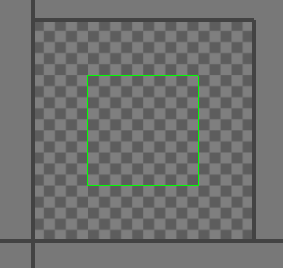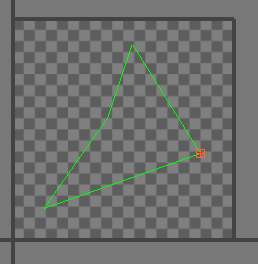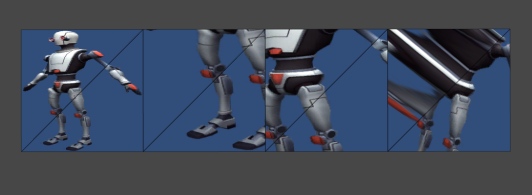float4 worldPos = mul(unity_ObjectToWorld,float4(0,0,0,1));
float2 bias = v.uv.xy *2-1;
worldPos.xyz += normalize(UNITY_MATRIX_V.xyz) * bias.r * 0.5+ normalize(UNITY_MATRIX_V.xyz)*bias.g * 0.5;

1.不能动态批处理。这个就不上图了

2.上一下测试模型234的图。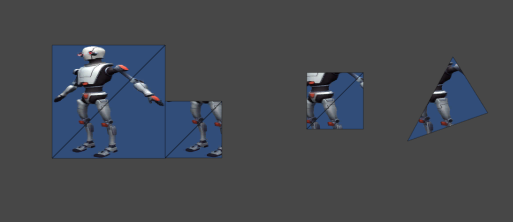float2 bias = v.vertex.xy*2;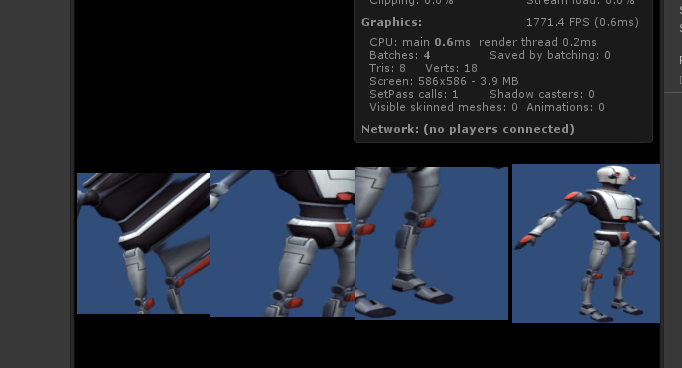-------------------------

-------------------------

float4 worldPos = mul(unity_ObjectToWorld,float4(0,0,0,1));
float2 bias = v.vertex.xy *2;
float3 forward = normalize(_WorldSpaceCameraPos- worldPos.xyz);
float3 up = normalize(UNITY_MATRIX_V.xyz);
float3 right = cross(forward,up);
worldPos.xyz += right * bias.r * 0.5+ up*bias.g * 0.5;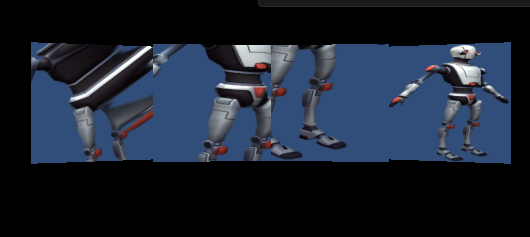float3 forward = normalize(_WorldSpaceCameraPos- worldPos.xyz);

o.pos = mul(UNITY_MATRIX_P,mul(UNITY_MATRIX_MV, float4(0, 0, 0, 1)) + float4(v.vertex.x, v.vertex.y, 0, 0)) ;